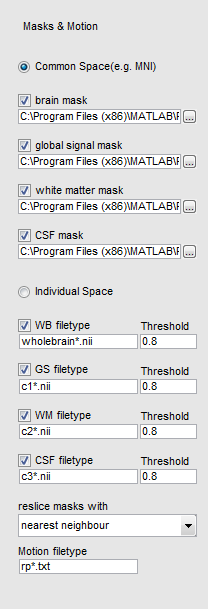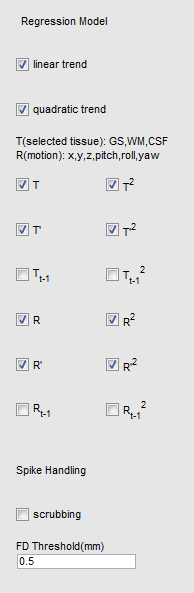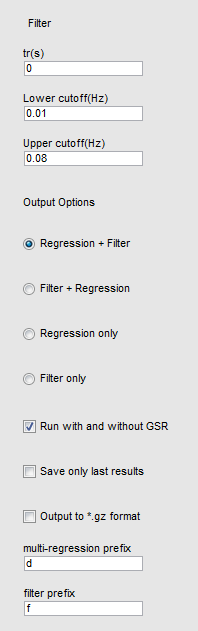# Denoise¶Input mask files for whole brain, global mean signal, white matter signal and CSF signal;

If common space is selected, one mask file for each mask type should be input.

If indivisual space is selected, wildcard for individual mask should be input, BRANT will search the mask within each subject’s directory.

The threshold for common space mask is 0.5 by default, while for individual space the threshold can be altered.

(common space masks normally are binarized, however in individual space are probability)

• reslice masks with: if the header information (size, FOV, originator, orientation and etc.) is different between data and mask, BRANT will: in common space reslice masks to the first input data

in individual space reslice masks to the each subject’s input data accordingly

If the masks are stored as binarized value, the suggested method for reslice is nearest neighbour, otherwise 4th degree B-spline.

• motion filetype: BRANT will search estimated headmotion file in each subject’s folder, normally by spm the file is `rp*.txt`• linear trend: regressor of 1:T
• quadratic trend: regressor of [1,22,32:T2]
• T: a gross regressors for selected global signal, white matter signal and CSF signal
• T2: element-wise square of T
• T’: temporal derivatives of T, zero padded
• T’2: element-wise square of T’
• Tt-1: 1-frame lagged T, zero padded
• Tt-1 2: element-wise square of Tt-1
• R: a gross matrix for motion, should be 6 columns, loaded from rp*.txt
• R2: element-wise square of R
• R’: temporal derivatives of R, zero padded
• R’2: element-wise square of R’
• Rt-1: 1-frame lagged R, zero padded
• Rt-1 2: element-wise square of Rt-1
• Spike Handling:

As suggested in 1. , spikes are censored with a threshold of FD.

FD is calculated as:

```motion_diff = diff(R);
FD = [0; sum([abs(motion_diff(:, 1:3)), 50 * abs(motion_diff(:, 4:6))], 2)];
```

The scrubbing will take place before the regression model. For example, if order of denoise is selected as Filter first and Regression, the scrubbing will not affect Filter.• Filter:
• tr: repetition time.
• lower cutoff (Hz): lower cutoff for band pass filter.
• upper cutoff (Hz): upper cutoff for band pass filter.
• Output Options:
• Regression + Filter: do regression first and then filter
• Filter + Regression: do filter first and then regression
• Regression only: do only regression
• Filter only: do only filter
• Run with and without GSR: if checked and mask of global signal is specified, BRANT will run selected process twice with and without global signal as regressor in T, and output to different file in the current and following processes.
• Save only last results: if checked, no middle files will be saved.
• Output to *.gz format: check to output to `*.gz` files (note that the following smooth process from SPM would require uncompressed files, if it’s checked, an error will occur in smooth); choolse if smooth is done beforehand or not required.
• multi-regression prefix: prefix for output of GLM
• filter prefix: prefix for output of filter
• References:
1. Power, J. D., Mitra, A., Laumann, T. O., Snyder, A. Z., Schlaggar, B. L., & Petersen, S. E. (2014). Methods to detect, characterize, and remove motion artifact in resting state fMRI. Neuroimage, 84(1), 320-341.